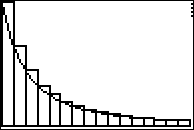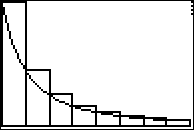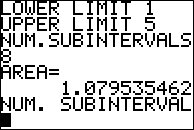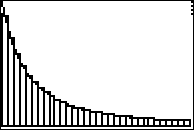# Activities

•• ##### Author50 Minutes

• ##### Other Materials
This is Activity 15 from the EXPLORATIONS Book:
Calculus Activities.

The following material is required for this activity:
• Graph Paper

## Approximating Integrals with Riemann Sums#### Activity Overview

In this activity, students calculate Riemann sums. They demonstrate when the Riemann sums will over-approximate a definite integral. They also observe the convergence of Riemann sums as the number of subintervals get larger.

#### Key Steps

•Students will calculate and analyze Reimann sums.

•Students will use a calculator program to automate the process of calculating the sum.

•Students will observe the convergence of left-hand and right-hand Riemann sums by using graphing
handhelds to automate the production of Riemann sums with regular partitions having a large number of subintervals.